# Examples

## Hand-Grab-Sphere-Reasoning

In the following we want to introduce you how AERA system learns to perform a simple task, involving a grabber (the agent’s body), a cube, and a sphere. The file `hand-grab-sphere.replicode` sets up the task. This example is a basic demonstration of how AERA models are used for planning and reasoning. The grabber grabs, moves and releases two objects. In this example, the goal of the task is to move the sphere s to position 15. Before the system starts performing the task, the cube c is attached to the hand h at position 25, while the sphere is at position 0. The commands that can be applied are move (limited to -/+ 20), grab and release. The following figure demonstrates the initial state of the task.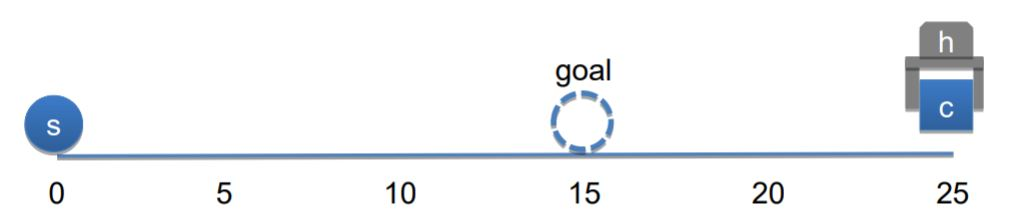### Seed Knowledge and Program

Seed program of AERA contains fundamental facts and knowledge about the task-environment that is given by the human programmer to the system. The knowledge includes basic causal models (e.g. grab object -> h attached object) and composite states based on which the models are instantiated. It also includes the high-level (root) goal, which is moving the sphere to the position 15 in this case. Seed also has programs by which the system starts bootstrapping its model generation (learning) process. In addition, some information about entities (e.g. hand h) and ontology classes (e.g. involved variables) are included.

### Reasoning Steps

The task-environment is composed of variables that might have different values at different times, depending on the applied command. For instance, if the move action is applied, the position of the hand (and the cube) changes in the next time step, which can be learned and used by the system as a causal model. But for using this model over inference steps, the system needs to have the composite state [h position P, c position P, h attached c] in the beginning, which means that it considers all relevant variables and their related values. In this example, a causal model connects a directly manipulable variable like grab, which belongs to the entity h, to an output variable which is attached. In other words, grabbing an object, causes that object to be attached to the hand. Now let us go through the reasoning steps of AERA when it performs the hand-grab-sphere task.

#### Steps 1 and 2: Move Hand to Sphere

AERA creates sub-goals and does backward chaining to perform the task. Since the high-level (root) goal is to release sphere at position 15, the system needs to make a sub-goal of hand being in the sphere’s position as the first step. So, it applies a move command to move towards where the sphere is, but due to the fact that I/O device has physical limitation, the hand will be at position 5 in the next frame. So, the same subgoal is created again for the next frame.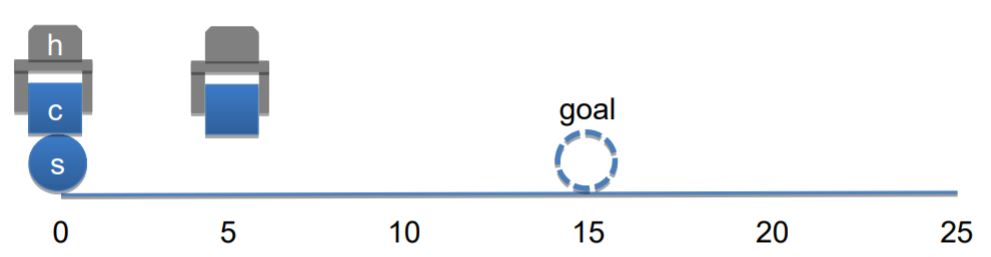#### Steps 3 and 4: Release Hand and Grab Sphere

Now that hand is reached to the position of sphere, AERA releases the cube, since that is a condition for grabing a new object, which is a sphere in this case. So it creates another causal model in the form of release h -> h attached nil, which means that if release action is taken the hand will be attached to nothing. AERA over its simulation process finds out that if the hand is empty, then the system can grab the sphere and move it to the goal position. In the fourth step, now that conditions are met, it creates the subgoal of making the hand attached to sphere, through an abduction which is done via causal model grab h -> h attached obj.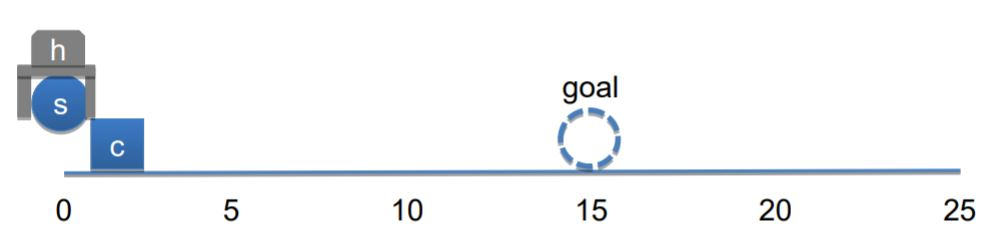#### Steps 5: Move Hand to Goal Position

Now that conditions for moving the sphere are met, the system uses the causal model move h DeltaP -> h position P+DeltaP, and the root goal is achived.

## Hand-Grab-Sphere-Learning

Now we go over how AERA system learns in `hand-grab-sphere-learn.replicode`. In this example, AERA learns from failing to grab the sphere s when the hand h releases the cube c at where the sphere is. In other words, since it fails to grab the sphere when both objects are at the same position it needs to learn to release the cube somewhere else and then grab the cube.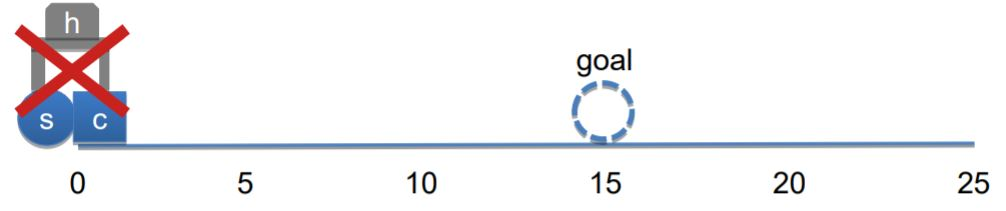In this situation, AERA starts guessing why its model M: grab h -> attached object fails. It then learns a meta-model which says that the model M would fail under specific conditions (i.e. when two objects are at the same position). Then, the system goes into babbling mode, in which it stops injecting the root goal and starts applying motor babbling commands (i.e. repeated release and grab) with the purpose of filtering out wrong requirement models (e.g. model M under the condition that there is only the sphere at where the hand is) and testing guessed causal models.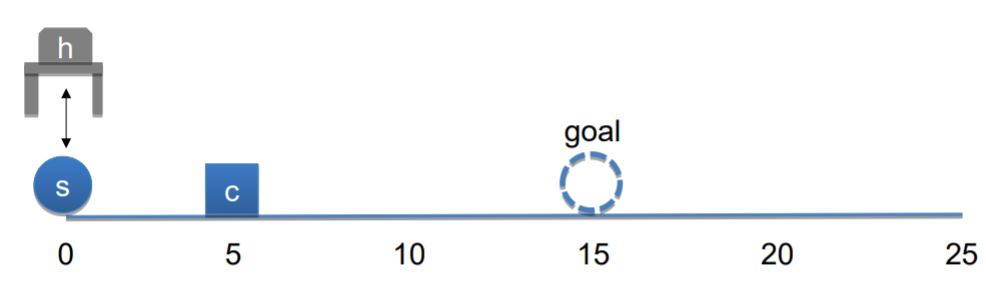Then, when a sufficient number of commands are applied, a new model is learned that allows grab h -> attached s with new conditions, after which it starts injecting the root goal again to make the system perform the task with new correct models. After resetting the task-environment to the initial state, the simulation process produces different sets of steps (simulation branches) of performing the task through abduction, in one of which the first action is to release the cube (in hand-grab-sphere-reasoning example the first action was to move).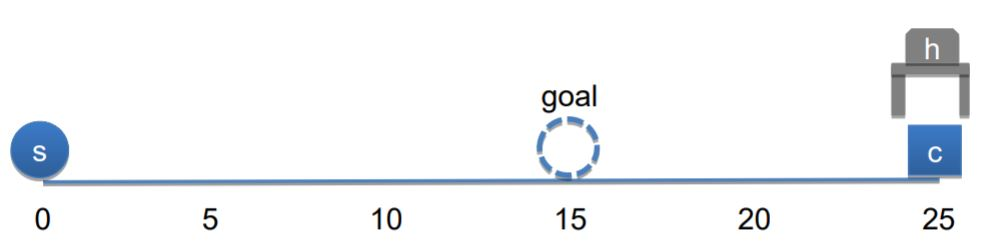Now the system is committed to releasing the “first release” branch, and thus the reasoning steps of performing the task becomes different. It releases the cube (step 1), moves towards where the sphere is (steps 2 and 3), grabs the sphere (step 4), and moves that to the goal position (step 5).

## Run the example

The according replicode files can be found in the source code of AERA:

``````.../AERA/AERA/replicode_v1.2/hand-grab-sphere.replicode
``````

and

``````.../AERA/AERA/replicode_v1.2/hand-grab-sphere-learn.replicode
``````

or in the GitHub repository here and here Change the `settings.xml` file in the AERA source code:

1. (If not already done) Set the parameter `keep_invalidated_objects` to `"yes"`
2. Set the source file to `hand-grab-sphere-learning.replicode` or `hand-grab-sphere.replicode`.

Run AERA (without debugging).

## Visualize the behavior of AERA

Open the AERA visualizer as described earlier and select the settings.xml file used to run the example. The visualizer reads the file and enables you to step through the time of the task performance of AERA.